# Operations on Rational Numbers

Operations on Rational Numbers

You must have studied about operations on fractions.

Rational numbers are expressed in the form of fractions, but we do not call them fractions as fractions include only positive numbers, while rational numbers include both positive and negative numbers.

Let us look at the chart given below to understand rational numbers in relation to other numbers.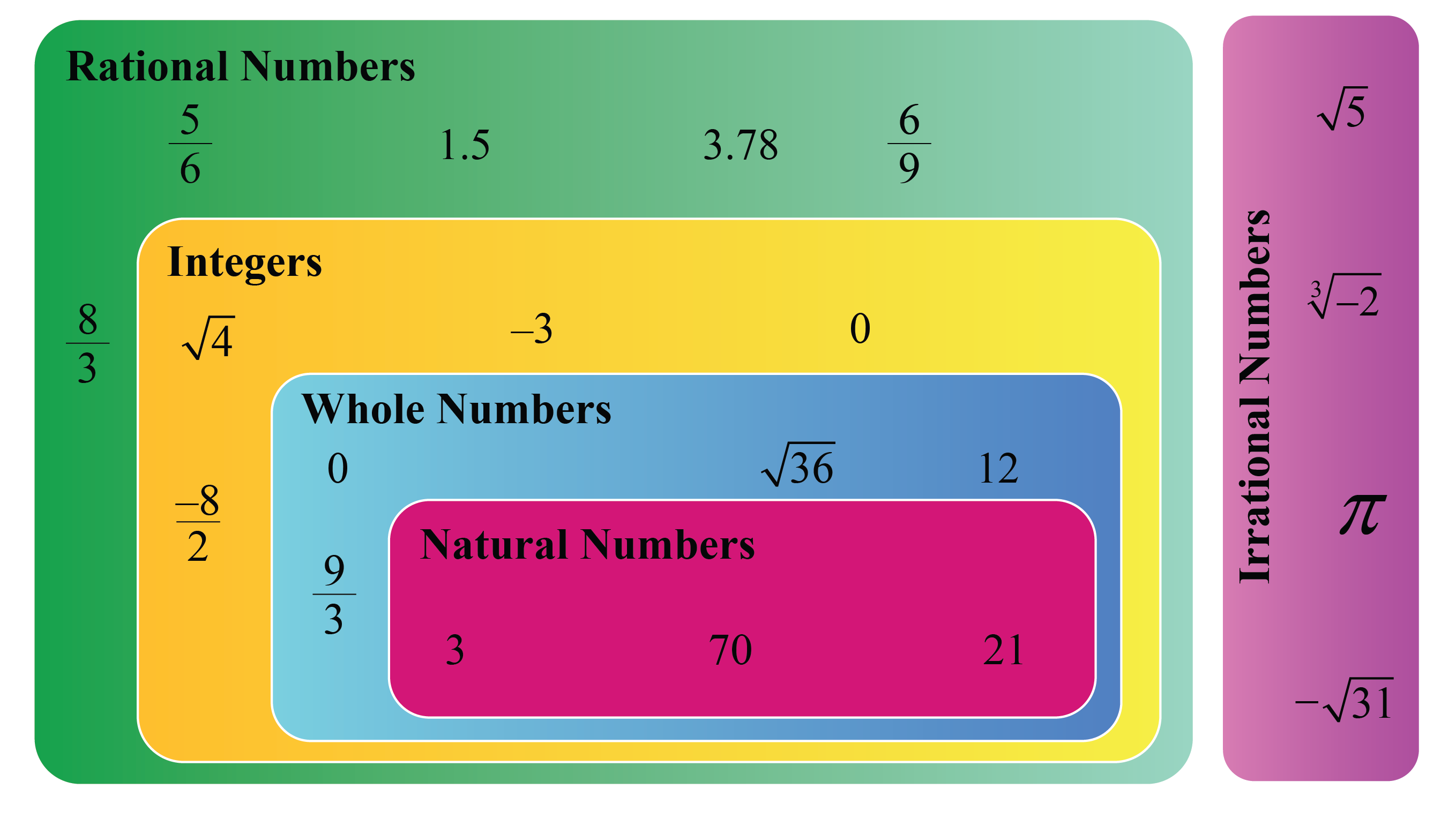Fractions are a part of rational numbers, while rational numbers is a broad category that includes other types of numbers.

Can we perform operations on rational numbers like how we do with fractions?

Let's find out!

In this mini-lesson, we will explore about operations on rational numbers by learning about addition, subtraction, multiplication, and division of rational numbers along with their properties.

Check out interesting simulations, solved examples, and interactive questions given at the end of the lesson.

## Lesson Plan

 1 What Are the Different Arithmetic Operations for Rational Numbers? 2 Important Notes 3 Solved Examples 4 Challenging Questions 5 Interactive Questions

## What Are the Different Arithmetic Operations for Rational Numbers?

A rational number is a number that is of the form $$\dfrac{p}{q}$$ where:

• $$p$$ and $$q$$ are integers
• $$q \neq 0$$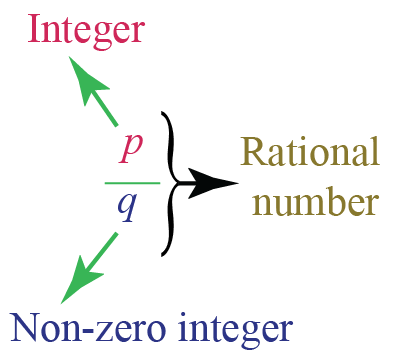Some examples o

f rational numbers are:

• $$\dfrac{1}{2}$$

• $$\dfrac{-3}{4}$$

• $$0.3$$ (or) $$\dfrac{3}{10}$$

• $$-0.7$$ (or) $$\dfrac{-7}{10}$$

You must know about fractions and how different operators can be used on different fractions.

All the rules and principles that apply to fractions can also be applied to rational numbers.

The one thing that we need to remember is that rational numbers also include negatives.

So, while $$\dfrac{1}{5}$$ is a rational number, it is true that $$\dfrac{-1}{5}$$ is also a rational number.

There are four basic arithmetic operations with rational numbers: addition, subtraction, multiplication, and division.

Let's learn about each in detail.

### Addition of Rational Numbers

Adding rational numbers can be done in the same way as adding fractions.

There are two cases related to addition of rational numbers.

• Adding rational numbers with like denominators
• Adding rational numbers with different denominators

To add two or more rational numbers with like denominators, we simply add all the numerators and write the common denominator.

For example, add $$\dfrac{1}{8}$$ and $$\dfrac{3}{8}$$.

Let us understand this with the help of a number line.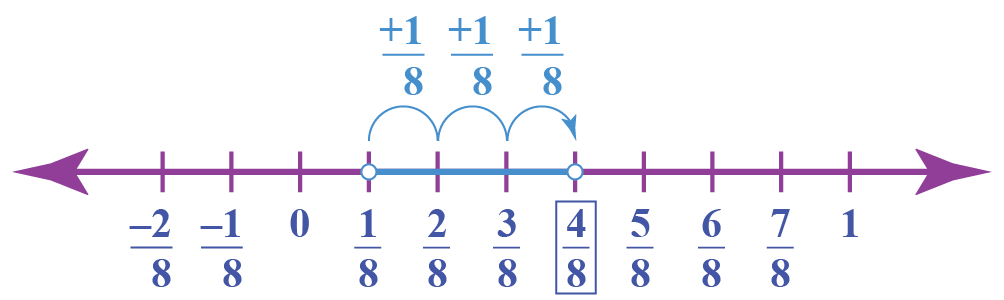On the number line, we start from $$\dfrac{1}{8}$$.

We will take $$3$$ jumps toward the right as we are adding $$\dfrac{3}{8}$$ to it.

As a result, we reach point $$\dfrac{4}{8}$$.

\begin{align}\ \frac{1}{8}+\frac{3}{8}=\frac{1+3}{8}\\ \end{align}

\begin{align}\ =\frac{4}{8} \end{align}

\begin{align}\ =\frac{1}{2} \end{align}

\begin{align}\ \therefore\frac{1}{8}+\frac{3}{8}=\frac{1}{2}\\ \end{align}

When rational numbers have different denominators, the first step is to make their denominators equivalent using the LCM of the denominators.

Let's consider an example.

Let us add the numbers \begin{align} \frac {-1}{3} \end{align} and \begin{align} \frac {3}{5} \end{align}

Step 1: The denominators are different in the given numbers.

Let's find the LCM of 3 and 5 to find the common denominator.

$$\text{LCM of 3 and 5} = 15$$

Step 2: Find the equivalent rational number with the common denominator.

To do this, multiply $$\dfrac{-1}{3}$$ with $$5$$ and $$\dfrac{3}{5}$$ with $$3$$

\begin{align} \dfrac{-1}{3} \times \dfrac{5}{5} = \dfrac{-5}{15} \end{align}

\begin{align} \dfrac{3}{5} \times \dfrac{3}{3} = \dfrac {9}{15} \end{align}

Step 3: Now the denominators are the same; simply add the numerators and then copy the common denominator.

Always reduce your final answer to its lowest term.

\begin{align} \dfrac {-1}{3} + \dfrac {3}{5} &= \left(\dfrac {-1}{3} \times \dfrac {5}{5}\right) + \left(\dfrac {3}{5} \times \dfrac {3}{3}\right) \\ &= \dfrac {-5}{15} + \dfrac {9}{15} \\ &= \dfrac {4}{15} \end{align}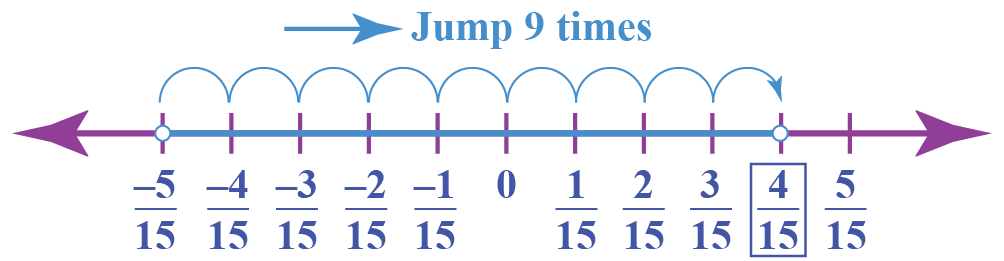### Subtraction of Rational Numbers

The process of subtraction of rational numbers is the same as that of addition.

While subtracting two rational numbers on a number line, we move toward the left.

Let us understand this method using an example.

Subtract $$\dfrac{1}{2}x-\dfrac{1}{3}x$$

Step 1- Find the LCM of the denominators.

LCM ($$2,3$$) = $$6$$

Step 2- Convert the numbers into their equivalents with $$6$$ as the common denominator.

$$\dfrac{1}{2}x \times \dfrac{3}{3}=\dfrac{3}{6}x$$

$$\dfrac{1}{3}x \times \dfrac{2}{2}=\dfrac{2}{6}x$$

Step 3- Subtract the numbers you obtained in step 2.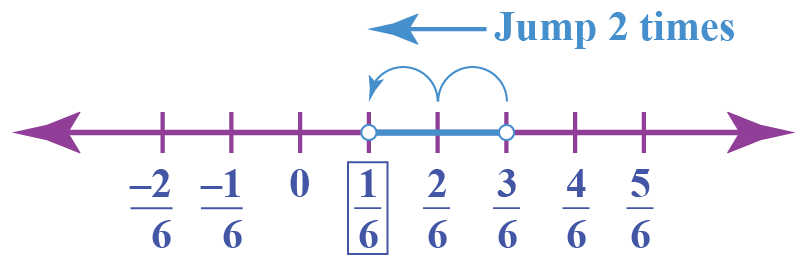\begin{align} \dfrac{3}{6}x-\dfrac{2}{6}x=(\dfrac{3-2}{6})x \end{align}

\begin{align} \ \ \ \ =\dfrac{x}{6} \end{align}

\begin{align} \therefore\dfrac{1}{2}x-\dfrac{1}{3}x=\dfrac{x}{6} \end{align}

### Multiplication of Rational Numbers

Multiplication of rational numbers is similar to how we multiply fractions.

To multiply any two rational numbers, we have to follow the steps given below:

1. Multiply the numerators
2. Multiply the denominators
3. Reduce the resulting number to its lowest term

Let's see how this is done with an example.

Let's multiply the following rational numbers:

\begin{align} \dfrac{-2}{3}\times(\dfrac{-4}{5}) \end{align}

The steps to find the solution are:

1. Multiply the numerators $$(-2)\times (-4)=8$$
2. Multiply the denominators $$3 \times 5=15$$
3. Since its already in its lowest term, we can leave it as is.

\begin{align} \dfrac{-2}{3}\times \dfrac{-4}{5} = \dfrac{(-2)\times (-4)}{3\times 5}= \dfrac{8}{15} \end{align}

### Division of Rational Numbers

We have learnt in whole number division that the dividend is divided by the divisor.

 \begin{align}\text{Dividend} \div \text{Divisor} = \frac{ \text{Dividend}}{\text{Divisor}}\end{align}

While dividing any two numbers, we have to see how many parts of the divisor are there in the dividend.

This is the same for the division of rational numbers as well.

The steps to be followed to divide two rational numbers are given below:

• Step 1: Take the reciprocal of the divisor (the second rational number)
• Step 2: Multiply it to the dividend
• Step 3: The product of these two numbers will be the solution.

Let us take an example to understand it in a better way.

\begin{align} \dfrac{-4x}{3}\div \dfrac{2x}{9} =\dfrac{-4x}{3} \times \dfrac{9}{2x}= -6 \end{align}

## What Are the Properties of Operations on Rational Numbers?

Some of the properties that apply to the operations on rational numbers are listed below:

 Meaning Property Closure Property This property states that when any two rational numbers are added, subtracted, multiplied or divided, the result is also a rational number. $$\dfrac{x}{y} \pm \dfrac{m}{n}=\dfrac{xn\pm ym}{yn}$$, which is a rational number. $$\dfrac{x}{y} \times \dfrac{m}{n}=\dfrac{xm}{yn}$$ $$\dfrac{x}{y} \div \dfrac{m}{n}=\dfrac{xn}{ym}$$ Associative Property For adding or multiplying three rational numbers, they can be rearranged internally without any effect on the final answer. This property does not hold true for subtraction and division of rational numbers. $$\dfrac{x}{y}+(\dfrac{m}{n}+\dfrac{p}{q})$$=$$(\dfrac{x}{y}+\dfrac{m}{n})+\dfrac{p}{q}$$ $$\dfrac{x}{y} \times (\dfrac{m}{n} \times \dfrac{p}{q})$$=$$(\dfrac{x}{y}\times \dfrac{m}{n}) \times \dfrac{p}{q}$$ Commutative Property This property states that two rational numbers can be added or multiplied irrespective of their order. This property does not hold true for subtraction and division of rational numbers. $$\dfrac{x}{y}+\dfrac{m}{n}=\dfrac{m}{n}+\dfrac{x}{y}$$ $$\dfrac{x}{y} \times \dfrac{m}{n}=\dfrac{m}{n} \times \dfrac{x}{y}$$ Additive/Multiplicative Identity $$0$$ is the additive identity of any rational number. When we add $$0$$ to any rational number, the resultant is the number itself. $$1$$ is the multiplicative inverse of any rational number. When we multiply $$1$$ to any rational number, the resultant is the number itself. $$\dfrac{x}{y}+0=\dfrac{x}{y}$$ $$\dfrac{x}{y} \times 1=\dfrac{x}{y}$$ Additive/Multiplicative Inverse For any rational number $$\dfrac{x}{y}$$, there exists  $$-\dfrac{x}{y}$$ such that the addition of both the numbers gives $$0$$.  $$-\dfrac{x}{y}$$ is the additive inverse of  $$\dfrac{x}{y}$$. Similarly, for any rational number $$\dfrac{x}{y}$$, there exists $$\dfrac{y}{x}$$ such that the product of both the numbers is equal to $$1$$. $$\dfrac{y}{x}$$ is the multiplicative inverse of $$\dfrac{x}{y}$$. $$\dfrac{x}{y}+(-\dfrac{x}{y})=0$$ $$\dfrac{x}{y} \times \dfrac{y}{x}=1$$ Distributive Property Two rational numbers combined with the addition or subtraction operator can be multiplied to a third rational number separately by putting the addition or subtraction sign in between. If there are $$3$$ rational numbers, $$\dfrac{p}{q}$$, $$\dfrac{m}{n}$$ and $$\dfrac{a}{b}$$, then, $$\dfrac{p}{q} \times (\dfrac{m}{n}\pm \dfrac{a}{b})$$=$$(\dfrac{p}{q} \times \dfrac{m}{n})\pm(\dfrac{p}{q} \times \dfrac{a}{b})$$

## Rational Numbers Calculator

You can add and subtract rational numbers on the calculator given below.

Enter any two rational numbers and click on the operator that you want to apply.

More Important Topics
Numbers
Algebra
Geometry
Measurement
Money
Data
Trigonometry
Calculus